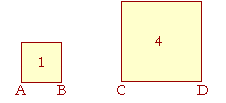The Evolution of the

R E A L  N U M B E R S

10

# SQUARESAND THEIR SIDES

The theorem of common measure

THESE ARE the first ten square numbers:

 1 4 9 16 25 36 49 64 81 100 And these are their roots: 1 2 3 4 5 6 7 8 9 10
 Problem 1.   What number is the square root of 25 144 ?

 5 12

Square unitsBy "1 square foot" we mean a square figure in which each side is 1 foot. 1 square foot is an area.  That is what kind of a magnitude it is. (Topic 7.)

And so, if 1 foot is the unit of length, then 1 square foot will be the unit of area.  If 1 centimeter is the unit of length, then 1 square centimeter is the unit of area. And so on.Look at this square whose side is 3 inches.  (By the "side" of a square, we mean any one of the four sides.)

Now, the side of the small shaded square is 1 inch.  The square itself therefore is 1 square inch.  And we can see that the entire square is made up of 9 of them.  The entire square is 9 square inches.

In other words, if the side of a square is N inches, then the square itself is N × N square inches.

Problem 2.

a)   The side of a square is 6 feet.  How big is the square itself?

36 square feet

b)   The side of a square is 4 meters.  How big is the square?

16 square meters

Problem 3.

a)   If you have a box of square tiles, all the same size, is it possible to

form a square figure from them by using only 3 tiles?   No.

b)  Could you form a square using 30 tiles?   No.

c)  What numbers of tiles must you use to form a square?

A square number.a)   The square drawn on AB is 25 square inches.  (AB is the side of the
a)   square.)  How long is AB itself?

5 inches

b)  What ratio does AB have to 1 inch?

AB : 1 inch = 5 : 1

c)  What ratio has the square on AB to 1 square inch.

Square on AB : 1 square inch = 25 : 1

Problem 5.    A square is composed of 49 square centimeters.  How long is the side?   7 centimeters
 Problem 6.   A square is made up of 6¼, or 25 4 , square centimeters.

How long is the side?

 52 or 2½  centimeters.

Common measure

Theorem.   Two straight lines will have a common measure -- they will have the same ratio as two natural numbers -- if and only if the squares on them have the same ratio as two square numbers.

The following problems are meant to illustrate that.

Problem 7.   E, AB, and CD are straight lines, and E is their common measure.  It measures AB four times and CD five times.

a)  Illustrate that.b)  What ratio has AB to CD?

AB : CD = 4 : 5

c)  What ratio has the square drawn on AB to the square drawn on CD?

Square on AB : Square on CD = 16 : 25

Problem 8.

a)  One straight line is twice as long as another.  Explicitly, in what ratio
a)  are the squares on them?The larger square is four times the smaller square.

b)  One straight line is three times another.  In what ratio are the squares
a)   on them?

The larger square is nine times the smaller square.

Problem 9.

a)   One square is to another as 25 is to 64.  Do their sides have a
a)   common measure?Yes. 25 and 64 are square numbers.

b)  Explicitly, in what ratio are their sides?

The smaller side is five eighths of the larger side.

c)  If the smaller side is 45 inches, then how long is the larger side?

Smaller side : Larger = 5 : 8 = 45 : 72.
The larger side is 72 inches.

Problem 10.   The square on AB is a fourth of the square on CD.a)  Do AB, CD have a common measure?

Yes. 1 and 4 are square numbers.

b)  If AB is 12 cm, how long is CD?

AB : CD = 1 : 2 = 12 : 24
CD is 24 cm.

Problem 11.   The square on AB is nine sixteenths of the square on CD.

a)  Do their sides have a common measure?Yes. 9 and 16 are square numbers.

b)  What ratio has AB to CD?

AB : CD = 3 : 4

c)  If CD is 1 inch, what length is a common measure of the sides?

¼ inch

d)  If AB is 1 inch, what length is a common measure of the sides?

1/3 inch

Problem 12.   Two squares are 20 square meters and 125 square meters.  Do their sides have a common measure?

20 : 125 = 4 : 25, upon dividing each term by 5.
The squares are therefore in the same ratio as square numbers. Therefore their sides have a common measure.

*

Now, what will be the case if two squares are not in the same ratio as two square numbers?  What if one square is twice the size of another?2 and 1 are not both square numbers.  What must we say about the ratio of the sides?  The answer is the subject of the next Lesson.

The theorem of this Lesson is credited to Theaetatus ("The-AY-ti-tus") of Athens, who was born around 417 B.C. There is a story recorded by Plato in his dialogue Theaetatus, that when Theaetatus was a boy, his teacher Theodorus brought him to meet Socrates. It was then that Socrates suggested to the youngster that it is not necessary to be intelligent, only to want to know.Next Topic:  Incommensurable magnitudes

Please make a donation to keep TheMathPage online.
Even \$1 will help.